# Clothes

CZK 2,400 cost clothes, a sweater by 150% than a T-shirt, trousers 2x more than a sweater, a jacket as a sweater and trousers together. What did the individual clothes cost?

Correct result:

b =  1125
n =  750
s =  375
t =  150

#### Solution:

2400 = s+t+n+b
s = t + 1.50t
n = 2s
b=s+n

2400 = s+t+n+b
s = t + 1.50•t
n = 2•s
b=s+n

b+n+s+t = 2400
s-2.5t = 0
n-2s = 0
b-n-s = 0

b = 1125
n = 750
s = 375
t = 150

Our linear equations calculator calculates it.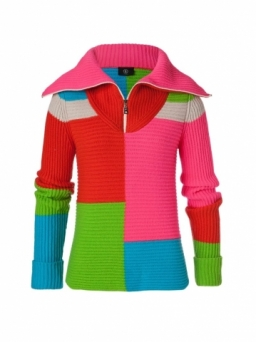We would be pleased if you find an error in the word problem, spelling mistakes, or inaccuracies and send it to us. Thank you!Tips to related online calculators
Do you have a system of equations and looking for calculator system of linear equations?

## Next similar math problems:The business earned CZK 98,400 in the shop in three weeks. In the second week, sales were 20% higher than the first week. The third week was 10% lower than the second week. How much did business earn in each week?
• Suit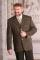The suit cost 119 euros. How much cost the pants if it is 50% cheaper than the jacket.
• Three workers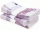The three workers received € 2,850 together for the work done. They divided them according to the time worked so that the first received 20% less than the second and the third € 50 more than the second. How much EUR did each worker receive?
• ContestantsThe three best contestants are to divide the total prize of CZK 4,200. The second gets 20% more than the third. And the first one gets 200 CZK less than the second and the third together. How much will everyone get?
• SavingsPaul has a by half greater savings than half Stanley, but the same savings as Radek. Staney save 120 CZK less than Radek. What savings have 3 boys together?
• Dividing moneyVilem, Cenek, and Edita divided the money they earned by spreading the leaflet. Vilem got 240 CZK more than Cenek and twice more than Edita. Edita got 400kc less than Vilem.
• Dividing moneyThomas, Honza and Vasek are to divide 1220kč. Honza got 25% more than Thomas. Vasek 20% less than Thomas. How CZK each got?
• Electricity consumption cost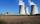Last year Karol family reduced its electricity consumption by 31% compared to the previous year and paid CZK 2883 less. How many CZK is electricity last year and how many two year ago?
• RewardThree workers have shared a common reward 13110 CZK follows: first worker got 35% less than the second and third worker got 20% more than the second worker. How much got each worker?
• Savings 2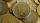Jozef and Michael saved 46 euros together. Michael saved 22 euros more than Jozef. How much did save each of them?
• Siblings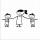Three siblings had saved up a total of 1,274 CZK. Peter had saved up to 15% more than Jirka and Hanka 10% less than Peter. How much money they saved each one of them?
• DiscountThe dress was discounted by CZK 115, which was 12% of the original price. What is the cost of the dress after the discount?
• RepairingThree employees earned a total of € 469 for repairing the equipment. They split so that the first got 20% more than the second, and the third 15% more than the second. How many euros did everyone get?
• Crowns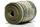1180 crowns is divided into three people that A got 20% less than B and C by 15% more than B. How many got A,B and C?
• EurosPeter, Jane and Thomas have together € 550. Tomas has 20 euros more than Jane, Peter € 150 less than Thomas. Determine how much has each of them.
• EmployeesThere are 1116 people working in three factory halls. In the first one, there are 18% more than the third, and 60 persons more than the second. How many employees work in individual halls?
• Jane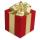Jane buy gift together for 464 Sk. For tie paid 4.5 times less than in the shirt, but 56 Sk more than for socks. How much she paid for each item in gift?